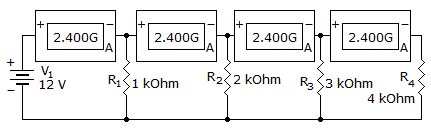# Electronics - Parallel Circuits - Discussion

Discussion Forum : Parallel Circuits - General Questions (Q.No. 10)
10.
Which component is shorted?R1
R2
R3
R4
Explanation:
No answer description is available. Let's discuss.
Discussion:
47 comments Page 1 of 5.

Man singh said:   9 years ago
'A' in the box of 2.400 G confirms that it is Galvanometer.

Now since ammeter is connected in series & zero resistance is assumed offered by ammeter. So while solving we can ignore/ discard the galvanometer(ammeter) & redraw the circuit & then Solve it.

Total I = 25mA.

I1 Across R1 = 12mA.
I2 Across R2 = 6mA.
I3 Across R3 = 4mA.
I4 Across R4 = 1mA.

Recheck Total I = (I1+I2+I3+I4) = 25mA;

Short circuit means more current flows; while open circuit means no current flows or less current flows.

Max current is flowing through R1 & less through R4.

Basically current is flowing though all Resistances. Also Rating of Galvanometer is 2.4 that is much higher than 25mA (Total current) hence all Galvanometer are in working condition again confirming that current is flowing through all Resistances.

I would like to conclude that R1 is comparatively short circuit. But we all are trying to justify R4 because R4 is the option given in the answer series.

Let me simplify this answer.... Look at the schematic and realize that "G" stands for conductance... So if you look at all of the branches, if either of the branches were to short other than R4, since current normally takes the easiest path, then there is no way that the current would be constant throughout every branch leading up to the final one in this circuit. So since R4 still has a the equivalent amount of the total conductance ( or current) then R4 would have to be the problematic component in this circuit.. This is a clear indication that current has taken the easiest pass at the very last branch of this circuit.

Here G stands for galvanometer itself. But 2.400G doesn't mean that the current in branch is 2.400GA. It just indicate the strength of galvanometer up to which it can measure the current. Since all the resistors are in parallel and the circuit acts as current divider more current flows from 1kohm and the rest is divide among others r4 gets less amount of current showing high resistance and low conductance. In general short circuit is nothing but the path for current having low impedance (high conductance) so the component which should be shorted should be r1.

Milongo said:   9 years ago
When you look at this circuit its shown that the current has only one path (as it is indicated by the Galvanometer). Now understanding the meaning of open circuit which could be the same as a burnt resistor (no current flow). Then by FORCE. R4 is going to get all the current. Making the path of R4 a SHORT circuit.

Now, This does not means that R4, is not there, yes is there and after some time it is going to burn. Since it is to much current going through. This is a problem. Puff fire all over. Poor resistor R4.

Prakash said:   10 years ago
Hey guys G is not a Conductance if its conductance means they denote it as (mho) not by G we have to use its unit only after the values not variables its galvanometer I agree wit pankaj he is right G is galvanometer and it measure current. 2.4 A is in the next G so no current through the R1. Similarly, no current through R2, R3 and finally whole current through R4. So R4 is short circuited and others are open circuit.

Mamta said:   7 years ago

As we all know, voltage is constant in parallel circuit. So I = V/R.

Current through R1 = 12 mA.

Similarly through R2, R3 and R4 is 6 mA, 4 mA and 3 mA.

Open circuit = No current or less current flows.

Short circuit = Large current flows.

But Large current flow is through. So how can R4 short circuit?

As per this rule R1 should be short circuited as low resistance is R1.

Vishnu said:   8 years ago
A galvanometer is a very sensitive meter which is used to measure tiny currents, usually 1 mA or less. And its symbol is different there is no image shows that current is passing through R4 or R4 act as a load better is to put a volt meter across every resistor to show that the current is passing through to simplify the question.

Sushama Saroj said:   7 years ago
Those of you who have optioned for R4, as the answer.

Here is my doubt for them as to why R4 was thought as shorted, and why not you guys said that any of the R1, R2 or R3 was shorted and the rest were open circuit.

Meaning, you guys could have said that R2 (or R1 or R3) was shorted, and the rest i, e R1, R3, and R4 were open.

Kevin said:   8 years ago
The way I made sense of this problem is to think of the battery as a voltage source with and internal series resistor not shown.

Short circuit current is 2.4 amps Internal series resistance can be calculated, R=I/V, R=2.4 amps/12 Volts = 0.2 ohms.

The only way to get all 4 ammeters to read 2.4 amps is for R4 to be shorted.